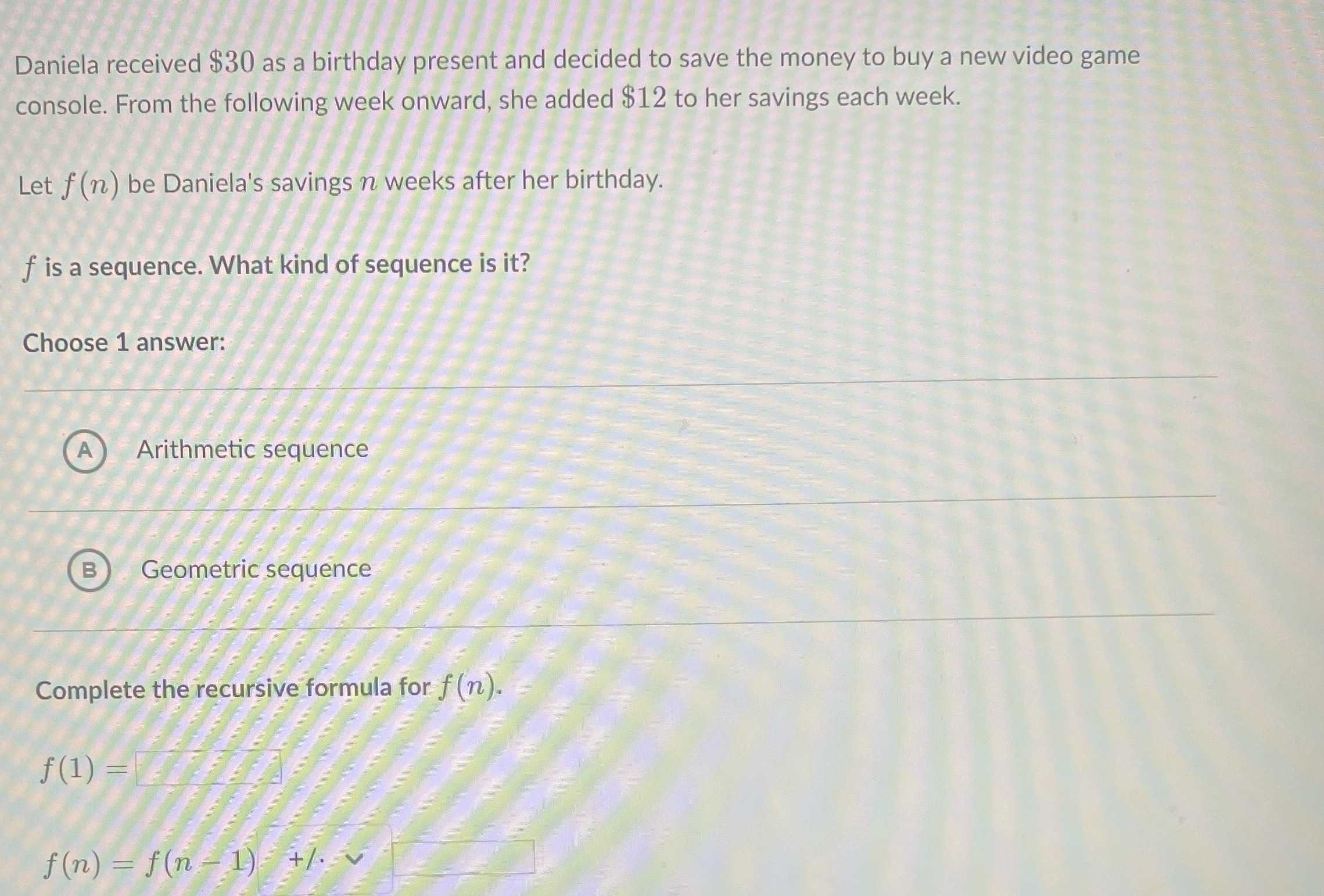### Still have math questions?

Algebra
QuestionDaniela received $$\ 30$$ as a birthday present and decided to save the money to buy a new video game console. From the following week onward, she added $$\ 12$$ to her savings each week.

Let $$f ( n )$$ be Daniela's savings $$n$$ weeks after her birthday.

$$f$$ is a sequence. What kind of sequence is it?

Choose $$1$$ answer:

A  Arithmetic sequence   B Geometric sequence

$$f ( 1 ) =$$_________

$$f(n)=f(n - 1 )$$________

$$f ( 1 ) = 42$$
$$f(n)=f(n - 1 )+12$$